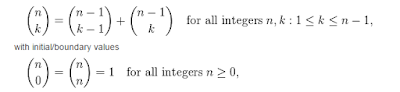## Wednesday, January 20, 2016

### Subsets and subgroups

[Attention, this code is not included in the code site!]

For finite sets there is a recursive method to compute the set of all subsets of a certain order which is an analogy to Pascals rule for binomial coefficients:From Wikipedia about binomial coefficient.
Suppose A is a set (with n elements for the analogy), that f is a choice function on all finite sets and that p(A,k) is the set of all subsets of A with k elements. For a set of sets S, define S%x to be the set consisting of all sets X in S where x have been included. Then

p(A,k)=p(A\{f(A)},k)+(p(A\{f(A)},k-1)%f(A))

where + stands for union of two disjoint sets: non of the sets in the first set include f(A), which every set in the second set do.

The choice function here is the topmost element on the stack where the set is pushed. This will work because the order of the sets in the computation not will be changed.

: nfence ( s -- s' )
ndup card nip 0 tuck
do >>yst fence yst>> nswap incl loop ;

The word nfence fence all the elements in the set s, for example:

{1,2,{3,4},0} --> {{1},{2},{{3,4}},{0}}

: power# ( s n -- s' )
>r ndup card r@ = if rdrop fence exit then
r@ 1 = if rdrop nfence exit then
nsplit obj>xst ndup r@ recurse
nswap r> 1- recurse xst>obj nincl union ;

3 sym 3 power# cr set.
{{132,321,231},{132,321,213},{321,231,213},{132,231,213},{132,321,312},{321,231,312},{132,231,312},{231,213,312},{321,213,312},{132,213,312},{132,321,123},{321,231,123},{132,231,123},{231,213,123},{321,213,123},{132,213,123},{213,312,123},{231,312,123},{321,312,123},{132,312,123}} ok

The next word make calculations for words computing subgroups and normal subgroups.

: presub ( s n -- s' )
>r nsplit >yst r> 1- power# yst> nincl ;

Using recursion has the disadvantage that it normally doesn't give partial outputs, but merely the whole output package in the end. The word psub# first use power# (in presub) to (try to) find the subsets of s and then tests which of the subsets that are groups. Since the identity permutation (at the top) must belong to every subgroup this is first excluded from s and then included in the end.

: psub# ( s n -- s' )
presub ndup card nip 0 tuck
do >>yst ndup pgr
if fence yst>> union
else ndrop yst>>
then
loop ;

4 alt 3 psub# cr set.
{{4132,2431,1234},{4213,3241,1234},{2314,3124,1234},{1342,1423,1234}} ok
4 alt 4 psub# cr set.
{{4321,2143,3412,1234}} ok

And pnsub# calculate the set of all normal subgroups of s with n elements:

: pnsub# ( s n -- s' )
>r >>xst nxst@ r> presub
ndup card nip 0 tuck
do >>yst ndup pgr
if ndup nxst@ pnormal
if fence yst>> union
else ndrop yst>>
then
else ndrop yst>>
then
loop nxstdrop ;

4 alt 2 pnsub# cr set.
0  ok
4 alt 3 pnsub# cr set.
0  ok
4 alt 4 pnsub# cr set.
{{4321,2143,3412,1234}} ok
4 alt 6 pnsub# cr set.
0  ok

The last do only works on GForth Androids and GForth 32 bit Windows. (SP-Forth if the stack is resized). It make overflow on the stack on the other systems. I'll had to make much more efficient routines, presumable with iteration instead of recursion.

Anyway, this shows that the group Alt(4), of order 12, only has one normal subgroup: {4321,2143,3412,1234} and only one quotient group:

4 alt ndup 4 pnsub# nunion pquote cr set.
{{4213,1342,2431,3124},{3241,4132,2314,1423},{4321,2143,3412,1234}} ok